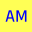AM
Animated Mathematics

###ContentVector addition and subtraction has many applications, such as descibing journeys on maps, and the addition of velocities and forces in physics.

### Vector Challenge

Read the text below and and then answer this question. Consider two vectors $$\mathbf{a}$$ and $$\mathbf{b}$$, these are added to calculate the vector $$\mathbf{c}$$ and subtracted to calculate the vector $$\mathbf{d}$$. For what special case is:

$$\lvert \mathbf{c} \rvert = \lvert \mathbf{d} \rvert$$ i.e. when the modulus (or the length) of the vectors are equal.

### click to reveal the answer

When the angle between vectors $$\mathbf{a}$$ and $$\mathbf{b}$$ is 90 degrees, a right angle.

The addition of vectors $$\mathbf{a}$$ and $$\mathbf{b}$$ can be visualized by drawing the vectors head to tail. The resultant vector is $$\mathbf{c}= \mathbf{a} +\mathbf{b}$$ . The x and y components are added together:

$$\mathbf{c}=\begin{bmatrix} 2\\ 1 \end{bmatrix} + \begin{bmatrix} 3\\ 4 \end{bmatrix} =\begin{bmatrix} 5\\ 5 \end{bmatrix}$$

The vector equation $$\mathbf{c}= \mathbf{a} +\mathbf{b}$$ resembles the addition of positive real numbers, but it must be appreciated that it is a vector equation where

$$\lvert \mathbf{c} \rvert \leqq \lvert \mathbf{a} \rvert + \lvert \mathbf{b} \rvert \$$

### Vector Subtraction

Subtracting vectors is almost the same procedure as addition, except the direction of the subtracted vector is reversed in direction. Here the same vectors are considered and we calculated the resultant vector labelled $$\mathbf{d}$$ where $$\mathbf{d}= \mathbf{a} -\mathbf{b}$$. We can also think of this as vector addition where the direction of $$\mathbf{b}$$ has been reversed, hence:

$$\mathbf{d}= \mathbf{a} +(\mathbf{-b} )$$ and the components are subtracted

$$\mathbf{D}=\begin{bmatrix} 2\\ 1 \end{bmatrix} - \begin{bmatrix} 3\\ 4 \end{bmatrix} =\begin{bmatrix} -1\\ 3 \end{bmatrix}$$

show

### We are going on a European Tour

content, equation example below

### Writing a vector equation

The vectors indicates the travel routes between two cities. We can write a vector equation for a journey by following a simple rule, we add the vector if we are travelling in the direction of the arrow, and subtract the vector when going in the opposite the arrow. For example the journey from Paris to London is summarised below:

Vector The Route
-b The most direct route via EuroStar
c-d A stop over at Berlin for shopping/seeing friends
c+e+f+g The Northern Tour via Sweden and Scotland
c+e-a via Berlin and Gothenburg

### Determine the possible vector equations for these journeys

#### Berlin to Aberdeen

There are no direct routes. -d-g via London, e+f via Gothenburg, e-a-g Gothenburg, London, and then Aberdeen.

#### London to Gothenburg

a is the direct flight. d+e a stopover at Berlin. -g-f via Aberdeen. b+c+e via Paris and Berlin.

#### Berlin to London

-d The direct flight. -c-b via Paris, e+f+g via Gothenburg and Aberdeen.